Win up to 100% scholarship on Aakash BYJU'S JEE/NEET courses with ABNAT Win up to 100% scholarship on Aakash BYJU'S JEE/NEET courses with ABNAT

# JEE Advanced Question Paper 2022 Chemistry Paper 1

JEE Advanced aspirants can access the JEE Advanced Question Paper 2022 Chemistry Paper 1 and solutions from this page. The best and the easiest approach is used by the specialists at BYJU’S to solve the question papers. Solving JEE Advanced previous years’ question papers will help the JEE aspirants understand the coverage of the topics and the pattern of the question paper. Candidates can evaluate their level of preparation by referring to the JEE Advanced 2022 Chemistry Paper 1 question paper and answer key provided here.

## JEE Advanced 2022 Chemistry Question Paper 1 with Solutions

SECTION – 1 (Maximum marks : 24)

∙ This section contains EIGHT (08) questions.

∙ The answer to each question is a NUMERICAL VALUE.

∙ For each question, enter the correct numerical value of the answer using the mouse and the on-screen virtual numeric keypad in the place designated to enter the answer. If the numerical value has more than two decimal places, truncate/round-off the value to TWO decimal places.

∙ Answer to each question will be evaluated according to the following marking scheme:

Full Marks : +3 ONLY if the correct numerical value is entered;

Zero Marks : 0 In all other cases.

1. 2 mol of Hg(g) is combusted in a fixed volume bomb calorimeter with excess of O2 at 298 K and 1 atm into HgO(s). During the reaction, temperature increases from 298.0 K to 312.8 K. If heat capacity of the bomb calorimeter and enthalpy of formation of Hg(g) are 20.00 kJ K–1 and 61.32 kJ mol–1 at 298 K, respectively, the calculated standard molar enthalpy of formation of HgO(s) at 298 K is X kJ mol–1. The value of |X| is _______.

[Given: Gas constant R = 8.3 J K–1 mol–1]

Sol. 2Hg(g) + O2(g) ⎯→ 2HgO(s)

Heat capacity of calorimeter = 20 kJ K–1

Rise in temperature = 14.8 K

Heat evolved = 20 × 14.8 = 296 kJ

ΔH° = ΔU° + ΔngRT

= –296 – 3 × 8.3 × 298 × 10–3

≃ –303.42 kJ

$$\begin{array}{l}\Delta H^\circ=\Delta H_f^o(HgO(s)) – \Delta H_f^o(Hg(g))\end{array}$$
$$\begin{array}{l} – 303.42 = \Delta H_f^o(HgO(s)) – 2 \times 61.32 \end{array}$$
$$\begin{array}{l}\Delta H_f^o(HgO(s)) = – 303.42 + 122.64 \\ = -180.78 ~\text{kJ}\end{array}$$
$$\begin{array}{l}|\,\Delta H_f^o(HgO(s))\,|\; = 90.39\,\,\text{kJ mol}{^{ – 1}} \end{array}$$

2. The reduction potential (E0, in V) of

$$\begin{array}{l}MnO_4^ – (aq)/Mn(s) \end{array}$$
is ______.

$$\begin{array}{l}[\text{Given}: E_{(MnO_4^-(aq)/MnO_2(s))}^0 = 1.68\ V;\ E_{(Mn{O_2}(s)/M{n^{2 + }}(aq))}^0 = 1.21\ V;\ E_{(M{n^{2 + }}(aq)/Mn(s))}^0 =-1.03\ V ]\end{array}$$

Sol. (1)

$$\begin{array}{l}MnO_4^-\left(aq\right)+4H^++3e\rightarrow MnO_2 \left(s\right)+2H_2O;E^\circ=1.68 \text{V}\end{array}$$
$$\begin{array}{l}\Delta G_1^o=- 3F\;(1.68) =-5.04\,\,F\end{array}$$

(2)

$$\begin{array}{l}MnO_2\left(s\right)+4H^++2e\rightarrow Mn^{2+}+2H_2O;E^\circ=1.21 \text{V}\end{array}$$
$$\begin{array}{l}\Delta G_2^o=-2F\;(1.21) =-2.42\ F\end{array}$$

(3)

$$\begin{array}{l}Mn^{2+}\left(aq\right)+2e\rightarrow Mn \left(s\right);E^\circ=-1.03 \text{V}\end{array}$$
$$\begin{array}{l}\Delta G_3^o=-2F(- 1.03)=+ 2.06\ F \end{array}$$

$$\begin{array}{l}MnO_4^-(aq)+8H^+ +7e\longrightarrow Mn(s)+4H_2O\end{array}$$
$$\begin{array}{l}\Delta G = \Delta G_1^o + \Delta G_2^o + \Delta G_3^o \end{array}$$

= (–5.04 – 2.42 + 2.06) F

–7F E° = –5.4F

E° = 0.77 V

3. A solution is prepared by mixing 0.01 mol each of H2CO3, NaHCO3, Na2CO3, and NaOH in 100 mL of water. pH of the resulting solution is _______.

[Given:
$$\begin{array}{l}p{K_{{a_1}}}\,\,and\,\,p{K_{{a_2}}} \end{array}$$
of H2CO3 are 6.37 and 10.32, respectively; log2 = 0.30]

Sol. First acid base reaction between H2CO3 and NaOH takes place.

In the final solution, we have 0.01 mole Na2CO3 and 0.02 moles of NaHCO3.

Here, we have a buffer of NaHCO3 and Na2CO3.

$$\begin{array}{l}\therefore pH = p{K_{{a_2}}} + \;\log \frac{{[Salt]}}{{[Acid]}} \end{array}$$
$$\begin{array}{l} = 10.32 + \log \;\frac{{\left( {\frac{{0.01}}{{0.1}}} \right)}}{{\left( {\frac{{0.02}}{{0.1}}} \right)}} \end{array}$$
$$\begin{array}{l} = 10.32 + \;\log \;\frac{1}{2} \end{array}$$

= 10.32 – log2

= 10.32 – 0.3

= 10.02

∴ pH = 10.02

4. The treatment of an aqueous solution of 3.74 g of Cu(NO3)2 with excess KI results in a brown solution along with the formation of a precipitate. Passing H2S through this brown solution gives another precipitate X. The amount of X (in g) is ________.

[Given: Atomic mass of H = 1, N = 14, O = 16, S = 32, K = 39, Cu = 63, I = 127]

Sol.

$$\begin{array}{l}\text{Number of moles of }Cu\left(NO_3\right)_2 =\frac{{3.74}}{{187}} = 0.02 \end{array}$$
$$\begin{array}{l}2Cu(NO_3)_2+4KI \to Cu_2I_2 \downarrow +I_2+ 4KNO_3 \end{array}$$
$$\begin{array}{l} \text{Number of moles of}~ Cu_2I_2 ~\text{precipitated} = 0.01\end{array}$$
$$\begin{array}{l}Cu_2I_2 +H_2S \rightarrow Cu_2S\downarrow +2HI\end{array}$$
$$\begin{array}{l}\text{Number of moles of} ~Cu_2S ~\text{precipitated} = 0.01\end{array}$$
$$\begin{array}{l}\text{Mass of}~ Cu_2S ~\text{precipitates} = \left(0.01 \times 158\right) g\\ = 1.58 g\end{array}$$

5. Dissolving 1.24 g of white phosphorous in boiling NaOH solution in an inert atmosphere gives a gas Q.
The amount of CuSO4 (in g) required to completely consume the gas Q is _______.

[Given: Atomic mass of H = 1, O = 16, Na = 23, P = 31, S = 32, Cu = 63]

Sol.

$$\begin{array}{l}[\underset{\begin{smallmatrix} 1.24\ g \\ or \\ 0.01\ mole \end{smallmatrix}}{\mathop{{{P}_{4}}}}\,+3NaOH+3{{H}_{2}}O\to P{{H}_{3}}+3Na{{H}_{2}}P{{O}_{2}}\]\end{array}$$

As NaOH is present in excess. So, amount of phosphine formed is 0.01 mole (as P4 is limiting)

$$\begin{array}{l}\underset{0.01\ mole}{2PH_3} + 3CuS{O_4} \to C{u_3}{P_2} + 3{H_2}S{O_4} \end{array}$$
$$\begin{array}{l}\text{Amount of}\ CuSO_4\ \text{required}=\frac{3 \times 0.01}{2}\ mole \end{array}$$
$$\begin{array}{l}\text{Mass of} CuSO_4 \left(\text{in g}\right)\text{required}= \frac{{0.03}}{2} \times (63 + 32 + 16\times 4) \end{array}$$
$$\begin{array}{l} = \frac{0.03}{2} \times 159 = 2.38\ \text{g} \end{array}$$

6. Consider the following reaction.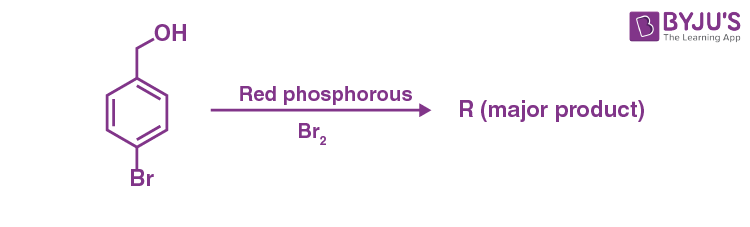On estimation of bromine in 1.00 g of R using Carius method, the amount of AgBr formed (in g) is ________.

[Given: Atomic mass of H = 1, C = 12, O = 16, P = 31, Br = 80, Ag = 108]

Sol. 2P + 3Br2 → 2PBr3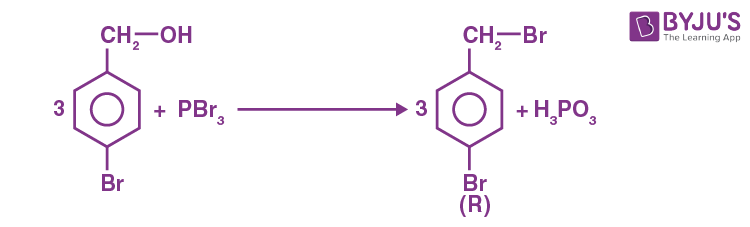Number of moles in 1 gm of (R) = 1/250

Number of moles of AgBr formed from (R) = 2/250

$$\begin{array}{l}\text{Mass of AgBr formed}=\frac{{2 \times 188}}{{250}} = 1.50~ \text{gm} \end{array}$$

7. The weight percentage of hydrogen in Q, formed in the following reaction sequence, is ________.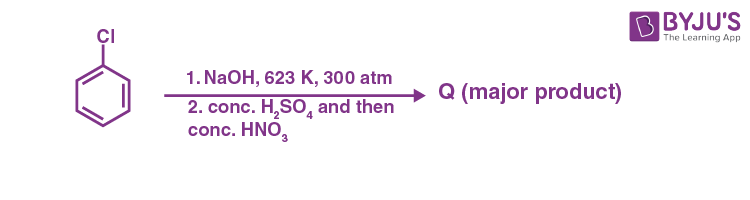[Given: Atomic mass of H = 1, C = 12, N = 14, O = 16, S = 32, Cl = 35]

Sol.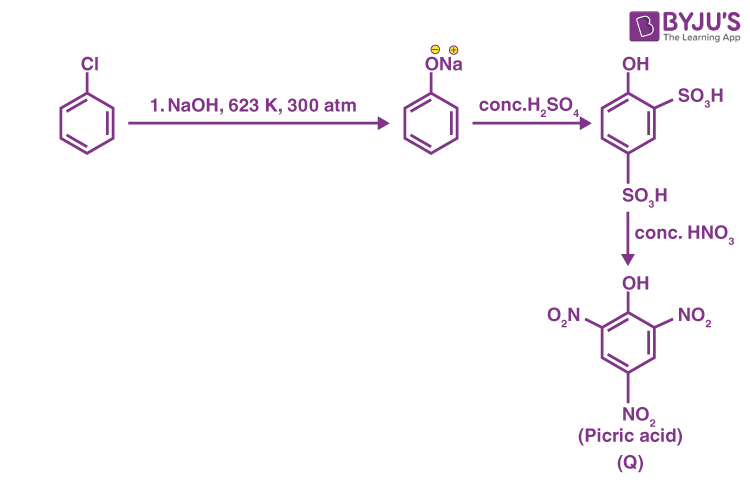Formula of compound = C6H3N3O7

Molar Mass of compound = (12 × 6 + 3 + 14 × 3 + 16 × 7) g

= 229 g

$$\begin{array}{l}\text{Weight}\ \% H =\frac{3}{{229}} \times 100 = 1.31 \end{array}$$

8. If the reaction sequence given below is carried out with 15 moles of acetylene, the amount of the product D formed (in g) is __________.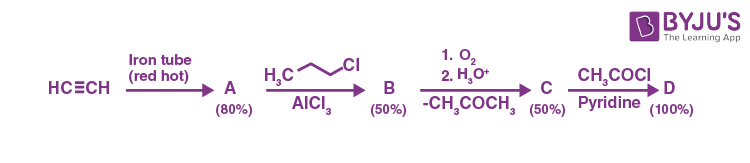The yields of A, B, C and D are given in parentheses.

[Given: Atomic mass of H = 1, C = 12, O = 16, Cl = 35]

Sol.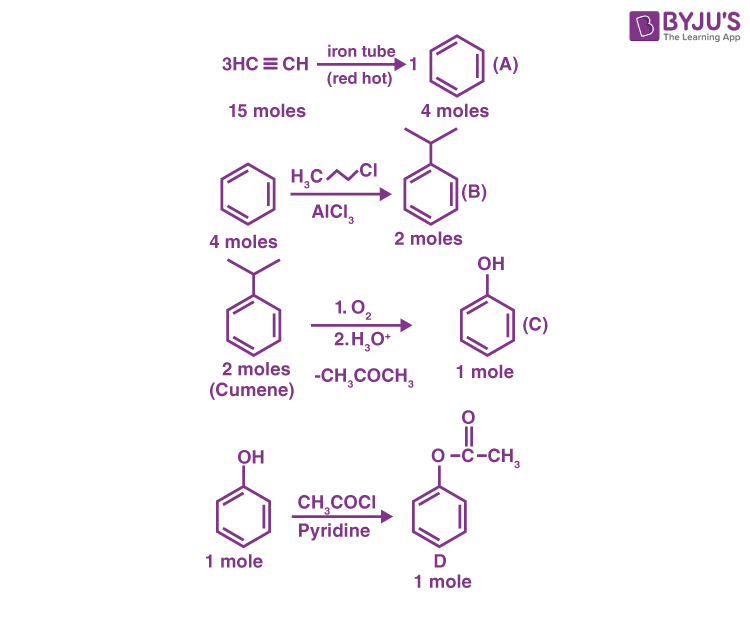Molecular formula of D is C8H8O2

Molar mass of D is (12 × 8 + 8 × 1 + 16 × 2) = 136 g

∴ Mass of D is 136

SECTION – 2 (Maximum marks : 24)

This section contains SIX (06) questions.

Each question has FOUR options (A), (B), (C) and (D). ONE OR MORE THAN ONE of these four option(s) is(are) correct answer(s).

∙ For each question, choose the option(s) corresponding to (all) the correct answer(s).

∙ Answer to each question will be evaluated according to the following marking scheme:

Full Marks : +4 ONLY if (all) the correct option(s) is(are) chosen;

Partial Marks : +3 If all the four options are correct but ONLY three options are chosen;

Partial Marks : + 2 If three or more options are correct but ONLY two options are chosen, both of which are correct;

Partial Marks : +1 If two or more options are correct but ONLY one option is chosen and it is a correct option;

Zero Marks : 0 If none of the options is chosen (i.e. the question is unanswered);

Negative Marks : –2 In all other cases.

9. For diatomic molecules, the correct statement(s) about the molecular orbitals formed by the overlap of two 2pz orbitals is(are)

(A) σ orbital has a total of two nodal planes.

(B) σ* orbital has one node in the xz-plane containing the molecular axis.

(C) π orbital has one node in the plane which is perpendicular to the molecular axis and goes through the center of the molecule.

(D) π* orbital has one node in the xy-plane containing the molecular axis.

Sol.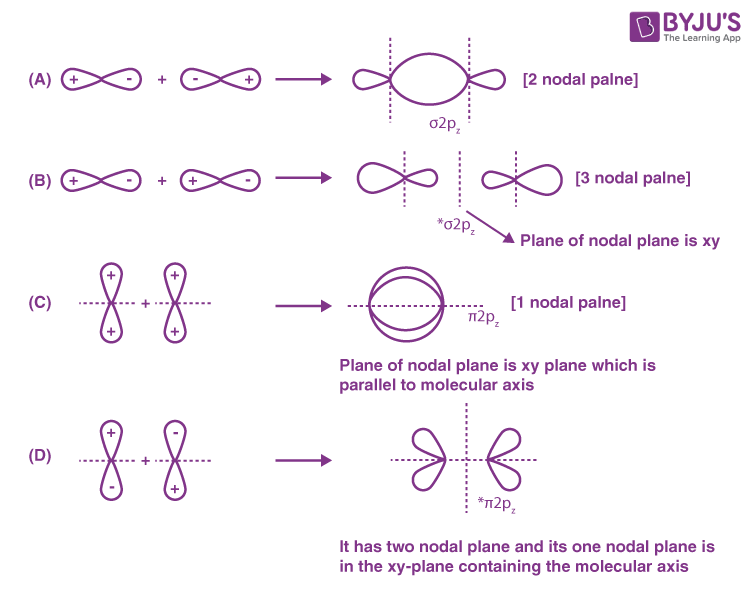10. The correct option(s) related to adsorption processes is(are)

(A) Chemisorption results in a unimolecular layer.

(B) The enthalpy change during physisorption is in the range of 100 to 140 kJ mol–1.

(C) Chemisorption is an endothermic process.

(D) Lowering the temperature favours physisorption processes.

Sol. (A) First statement is correct as chemisorption results in a unimolecular layer and physisorption result in a multimolecular layer.

(B) Second statement is incorrect as enthalpy change during physisorption is of the range of (20 – 40) kJ mol–1.

(C) Chemisorption is an exothermic process with (80 – 240) kJ mol–1 as the enthalpy of adsorption.

(D) Lowering the temperature results in increase in the extent of physisorption.

Hence (A) and (D) are correct.

11. The electrochemical extraction of aluminium from bauxite ore involves

(A) the reaction of Al2O3 with coke (C) at a temperature > 2500°C.

(B) the neutralization of aluminate solution by passing CO2 gas to precipitate hydrated alumina (Al2O3.3H2O).

(C) the dissolution of Al2O3 in hot aqueous NaOH.

(D) the electrolysis of Al2O3 mixed with Na3AlF6 to give Al and CO2.

Sol. (A) The reduction of Al2O3 with coke (C) at a temperature > 2500°C is not carried out due to the formation of carbides.

(B) It is correct as neutralisation of aluminate solution is done by passing CO2 gas to precipitate hydrated alumina.

(C) Reaction of powdered one is carried out with hot concentrated NaOH at 473 K – 523 K and 35 – 36 bar pressure.

(D) Electrolysis of Al2O3 is done mixed with Na3AlF6 to produce Al and CO2. It is a correct statement.

12. The treatment of galena with HNO3 produces a gas that is

(A) paramagnetic

(B) bent in geometry

(C) an acidic oxide

(D) colorless

Sol. PbS + dil. HNO3 → Pb(NO3)2 + S + NO + H2O

NO is paramagnetic due to the presence of unpaired electrons. It is a neutral oxide. It is colourless.

Hence, (A) and (D) are correct statements.

13. Considering the reaction sequence given below, the correct statement(s) is(are)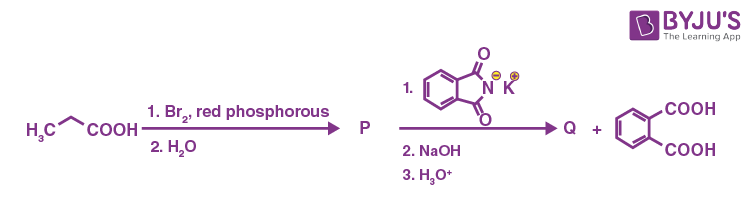(A) P can be reduced to a primary alcohol using NaBH4.

(B) Treating P with conc. NH4OH solution followed by acidification gives Q.

(C) Treating Q with a solution of NaNO2 in aq. HCl liberates N2.

(D) P is more acidic than CH3CH2COOH.

Sol.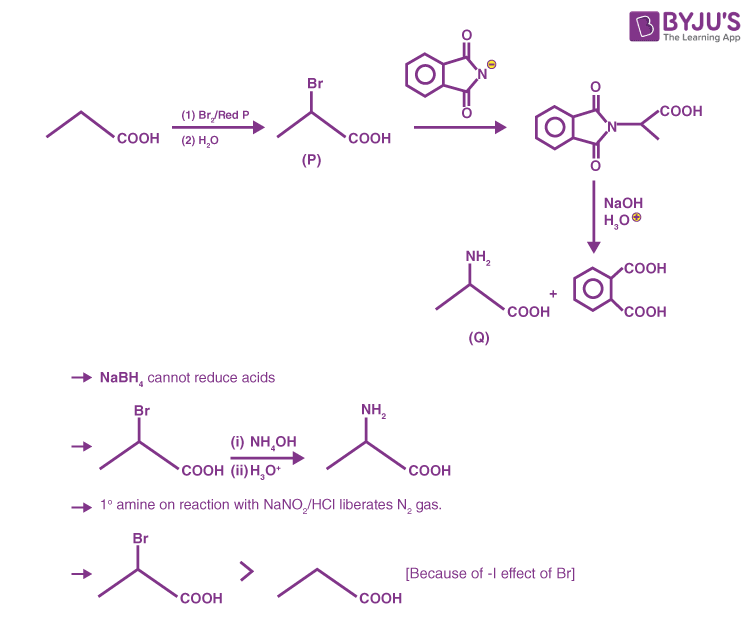14. Considering the following reaction sequence,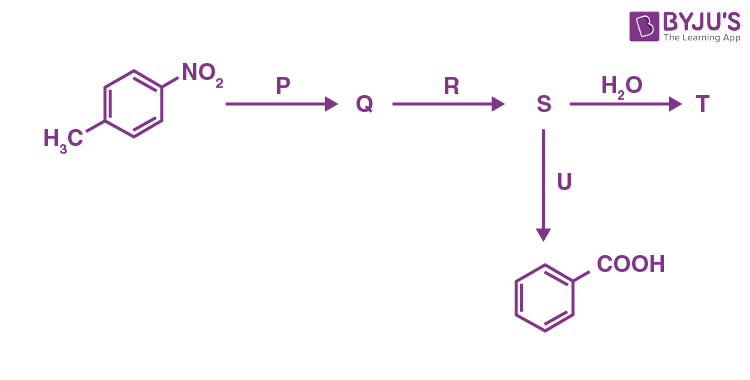the correct option(s) is(are)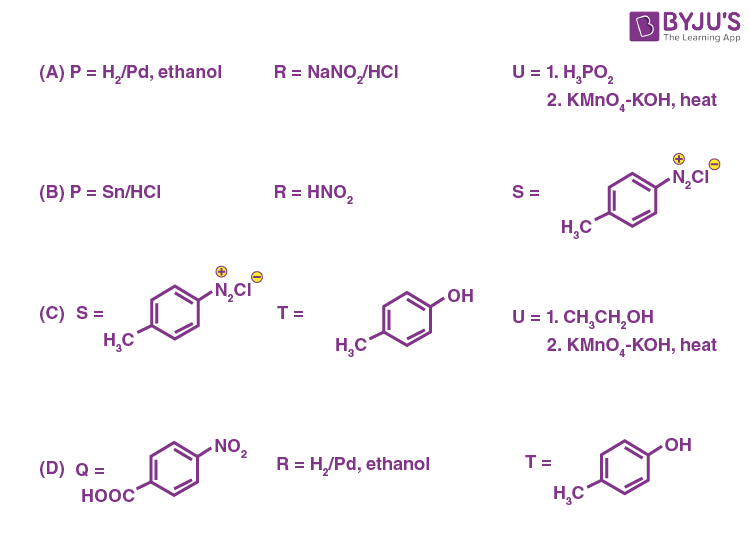Sol.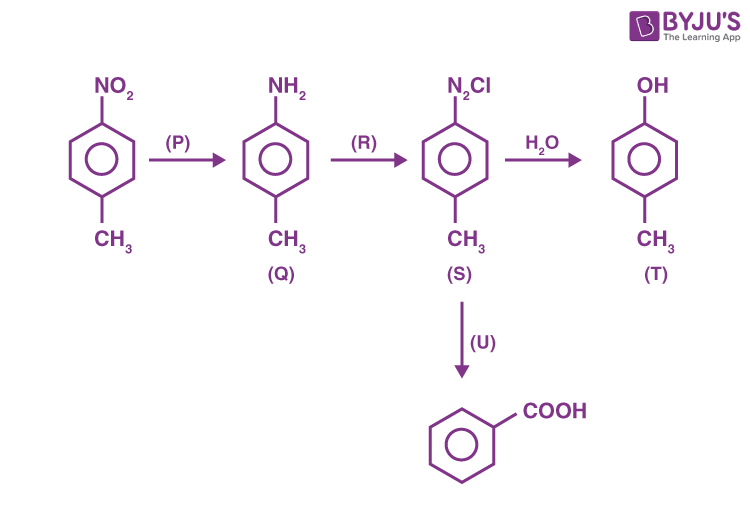→ P may be → H2/Pd, ethanol; Sn/HCl

→ R may be → NaNO2/HCl; HNO2

→ U may be → (i) H3PO2, (ii) KMnO4 – KOH, Δ or (i) CH3 – CH2 – OH, (ii) KMnO4 – KOH, Δ

SECTION – 3 (Maximum marks : 12)

∙ This section contains FOUR (04) Matching List Sets.

∙ Each set has ONE Multiple Choice Question.

∙ Each set has TWO lists: List-I and List-II.

List-I has Four entries (I), (II), (III) and (IV) and List-II has Five entries (P), (Q), (R), (S) and (T).

∙ FOUR options are given in each Multiple Choice Question based on List-I and List-II and ONLY ONE of these four options satisfies the condition asked in the Multiple Choice Question.

∙ Answer to each question will be evaluated according to the following marking scheme:

Full Marks : +3 ONLY if the option corresponding to the correct combination is chosen;

Zero Marks : 0 If none of the options is chosen (i.e. the question is unanswered);

Negative Marks : −1 In all other cases.

15. Match the rate expressions in LIST-I for the decomposition of X with the corresponding profiles provided in
LIST-II. Xs and k are constants having appropriate units.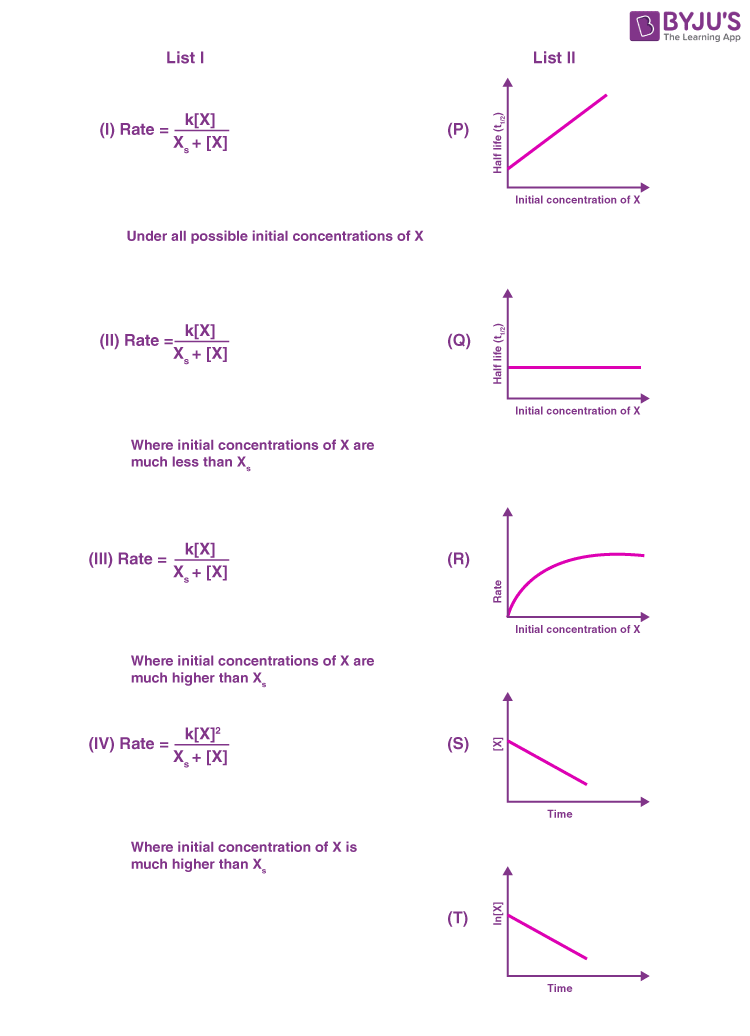(A) I→ P; II→ Q; III → S; IV → T

(B) I→ R; II→ S; III → S; IV → T

(C) I→ P; II→ Q; III → Q; IV → R

(D) I→ R; II→ S; III → Q; IV → R

Sol. (I)

$$\begin{array}{l}rate = \frac{{k\left[ X \right]}}{{{X_s} + \left[ X \right]}} \end{array}$$

Case-1: [X] >> Xs; [X] + Xs ≈ [X]

$$\begin{array}{l}rate = \frac{{k\left[ X \right]}}{{\left[ X \right]}}=k\left(\text{Zero order w.r.t. X}\right)\end{array}$$

I → P, S

Case-2: [X] << Xs; [X] + Xs ≈ Xs

$$\begin{array}{l}\therefore rate = \frac{{k\left[ X \right]}}{{{X_s}}} = k’\left[ X \right] \left(1^{st} \text{order w.r.t. X}\right)\end{array}$$

∴ I → Q, T

Case-3: [X] ≈ Xs

$$\begin{array}{l}rate = \frac{{k\left[ X \right]}}{{{X_s} + \left[ X \right]}} \end{array}$$

In this case curve-R given in List-II will match.

∴ I → P, Q, R, S, T (The graph of half-life should start from origin)

(II)

$$\begin{array}{l}rate = \frac{{k\left[ X \right]}}{{{X_s} + \left[ X \right]}} \end{array}$$

∵ [X] << Xs

∴ Xs + [X] ≈ Xs

$$\begin{array}{l}\therefore rate = \frac{{k\left[ X \right]}}{{{X_s}}} = k’\left[ X \right] \left(1^{st} \text{order w.r.t. X}\right)\end{array}$$

∴ II → Q, T

(III)

$$\begin{array}{l}rate = \frac{{k\left[ X \right]}}{{{X_s} + \left[ X \right]}} \end{array}$$

∵ [X] >> Xs

∴ Xs + [X] ≈ [X]

$$\begin{array}{l}\therefore rate = \frac{{k\left[ X \right]}}{{\left[ X \right]}} = k \left(\text{Zero order w.r.t. X}\right)\end{array}$$

∴ III → P, S

(IV)

$$\begin{array}{l}rate = \frac{{k{{\left[ X \right]}^2}}}{{{X_s} + \left[ X \right]}} \end{array}$$

∵ [X] >> Xs

∴ Xs + [X] ≈ [X]

$$\begin{array}{l}\therefore rate = \frac{{k{{\left[ X \right]}^2}}}{{\left[ X \right]}} = k\left[ X \right] \left(1^{st}~ \text{order w.r.t. X}\right)\end{array}$$

∴ IV → Q, T

16. LIST-I contains compounds and LIST-II contains reactions.

 LIST-I LIST-II (I) H2O2 (P) Mg(HCO3)2 + Ca(OH)2 → (II) Mg(OH)2 (Q) BaO2 + H2SO4 → (III) BaCl2 (R) Ca(OH)2 + MgCl2 → (IV) CaCO3 (S) BaO2 + HCl → (T) Ca(HCO3)2 + Ca(OH)2 →

Match each compound in LIST-I with its formation reaction(s) in LIST-II, and choose the correct option

(A) I → Q; II → P; III → S; IV → R

(B) I → T; II → P; III → Q; IV → R

(C) I → T; II → R; III → Q; IV → P

(D) I → Q; II → R; III → S; IV → P

Sol.

$$\begin{array}{l}\left(P\right) Mg\left(HCO_3 \right)_2 + 2Ca\left(OH\right)_2\rightarrow 2CaCO_3 \downarrow + Mg\left(OH\right)_2\downarrow + 2H_2O\\\left(Q\right) BaO_2 + H_2SO_4 \rightarrow BaSO_4 + H_2O_2\\\left(R\right) Ca\left(OH\right)_2 +MgCl_2 \rightarrow Mg\left(OH\right)_2 + CaCl_2\\\left(S\right) BaO_2 + 2HCI\rightarrow BaCl_2 + H_2O_2\\\left(T\right) Ca\left(HCO_3 \right)_2 + Ca\left(OH\right)_2 \rightarrow 2CaCO_3 \downarrow + 2H_2O\end{array}$$

I → Q

II → R

III → S

IV → P

Option (D) is correct.

17. LIST-I contains metal species and LIST-II contains their properties.

 LIST-I LIST-II (I) [Cr(CN)6]4– (P) t2g orbitals contain four electrons (II) [RuCl6]2– (Q) μ(spin-only) = 4.9 BM (III) [Cr(H2O)6]2+ (R) low spin complex ion (IV) [Fe(H2O)6]2+ (S) metal ion in 4+ oxidation state (T) d4 species

[Given: Atomic number of Cr = 24, Ru = 44, Fe = 26]

Match each metal species in LIST-I with their properties in LIST-II, and choose the correct option

(A) I → R, T; II → P, S; III → Q, T; IV → P, Q

(B) I → R, S; II → P, T; III → P, Q; IV → Q, T

(C) I → P, R; II → R, S; III → R, T; IV → P, T

(D) I → Q, T; II → S, T; III → P, T; IV → Q, R

Sol. (I) [Cr(CN)6]4–:

Cr2+ = [Ar] 3d4 4s0

It is d2sp3 hybridised as CNis a strong field ligand.

(II) [RuCl6]2–:

Ru4+ = [Kr] 4d4 5s0

t2g set contains four electron.

(III) [Cr(H2O)6]2+:

Cr2+ = [Ar] 3d4 4s0

It has four unpaired electrons as H2O is weak field ligand.

So, its μ = 4.9 B.M.

(IV) [Fe(H2O)6]2+:

Fe2+ = [Ar]3d6 4s0

$$\begin{array}{l}= t_{2g}^4 e_g^2 \end{array}$$

It has four unpaired electrons, its μ = 4.9 B.M.

18. Match the compounds in LIST-I with the observations in LIST-II, and choose the correct option.

 LIST-I LIST-II (I) Aniline (P) Sodium fusion extract of the compound on boiling with FeSO4, followed by acidification with conc. H2SO4, gives Prussian blue color. (II) o-Cresol (Q) Sodium fusion extract of the compound on treatment with sodium nitroprusside gives blood red color. (III) Cysteine (R) Addition of the compound to a saturated solution of NaHCO3 results in effervescence. (IV) Caprolactam (S) The compound reacts with bromine water to give a white precipitate. (T) Treating the compound with neutral FeCl3 solution produces violet color.

(A) I → P, Q; II → S; III → Q, R; IV → P

(B) I → P; II → R, S; III → R; IV → Q, S

(C) I → Q, S; II → P, T; III → P; IV → S

(D) I → P, S; II → T; III → Q, R; IV → P

Sol. (I) Aniline:Since it contains both carbon and nitrogen so its sodium fusion extract with boiling FeSO4, followed by acidification with conc. H2SO4 gives Prussian blue colour.

Fe2+ + 6CN → [Fe(CN)6]4–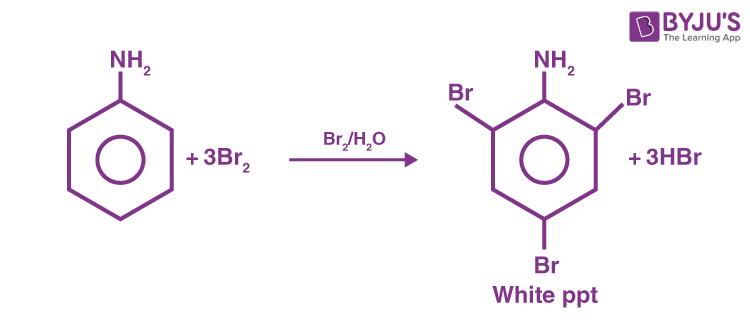I – (P, S)

(II) o-Cresol: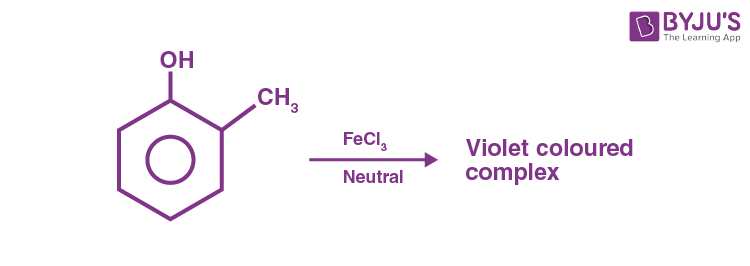(III) Cysteine:Since it has both, sulphur and nitrogen, so its sodium fusion extract will give blood red colour with Fe3+ and it has carboxylic group so it will give effervescence with NaHCO3.

(IV) Caprolactam:Its sodium fusion extract will give Prussian blue colour on boiling with FeSO4 followed by acidification with conc. H2SO4.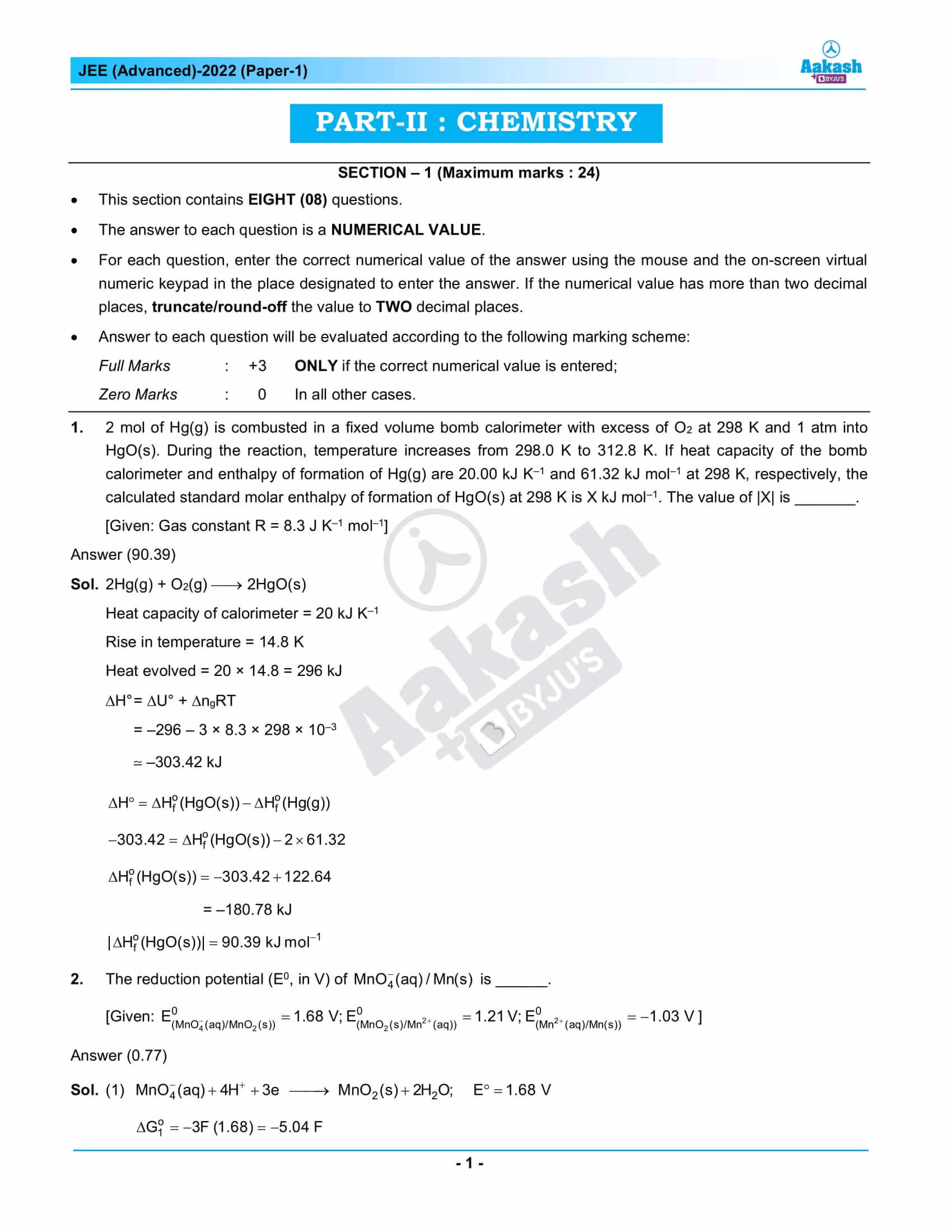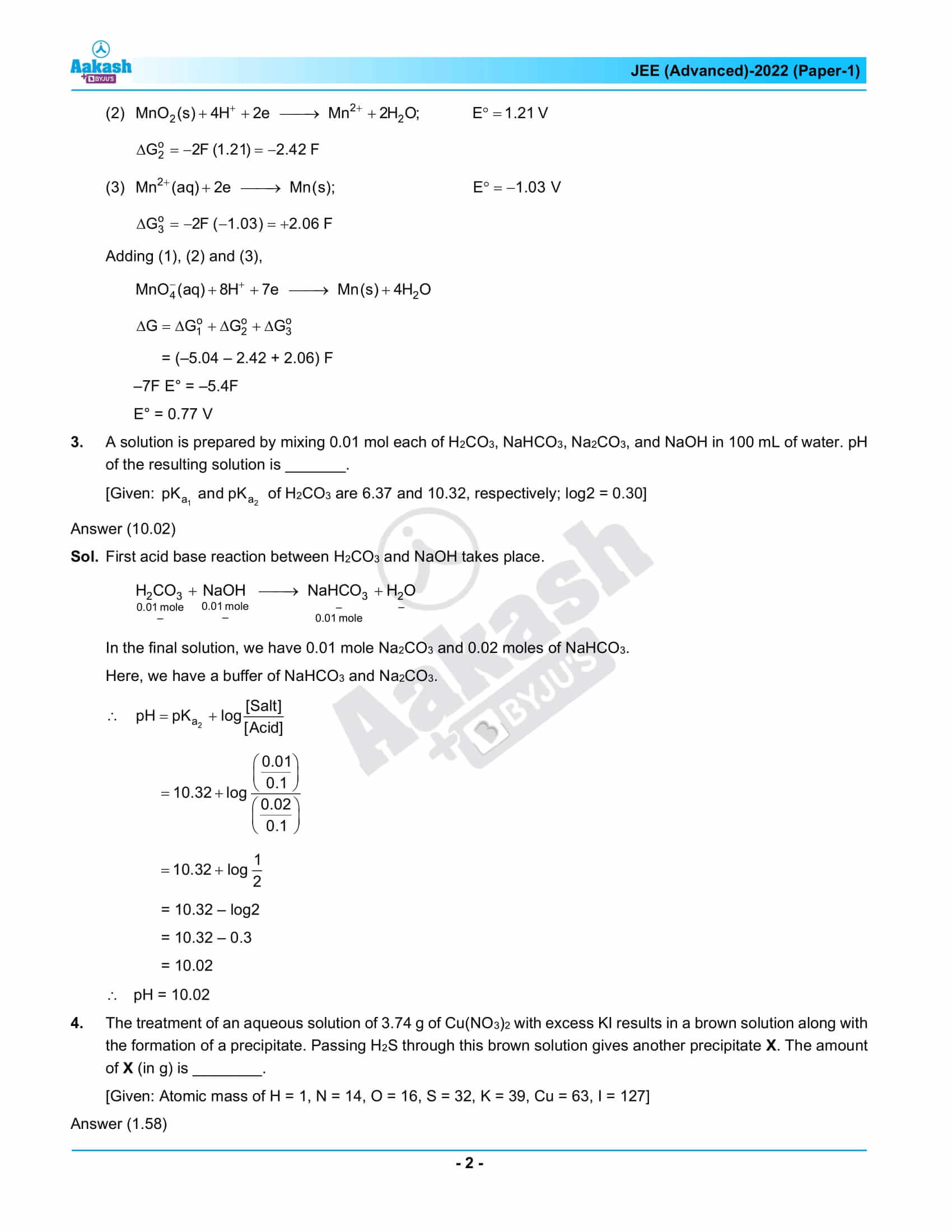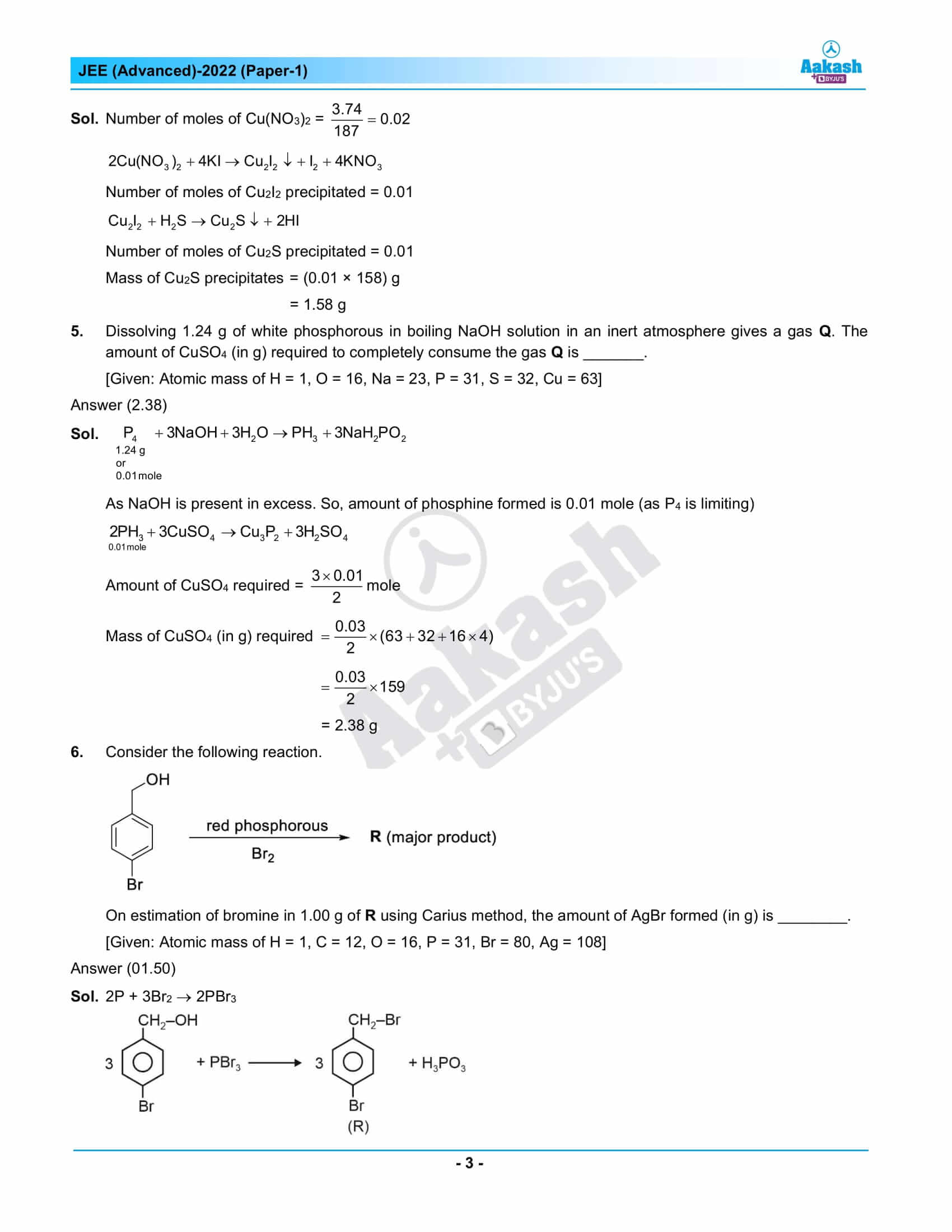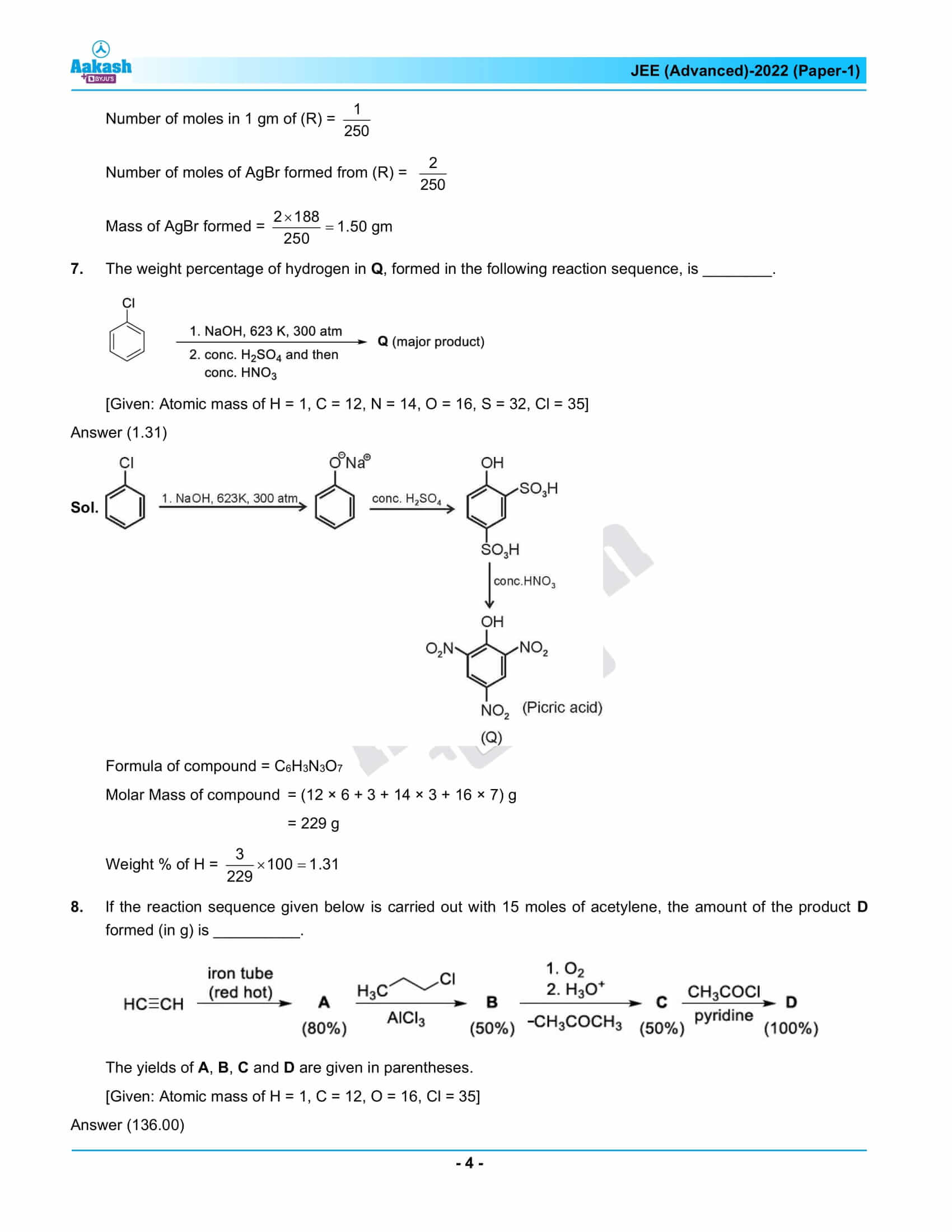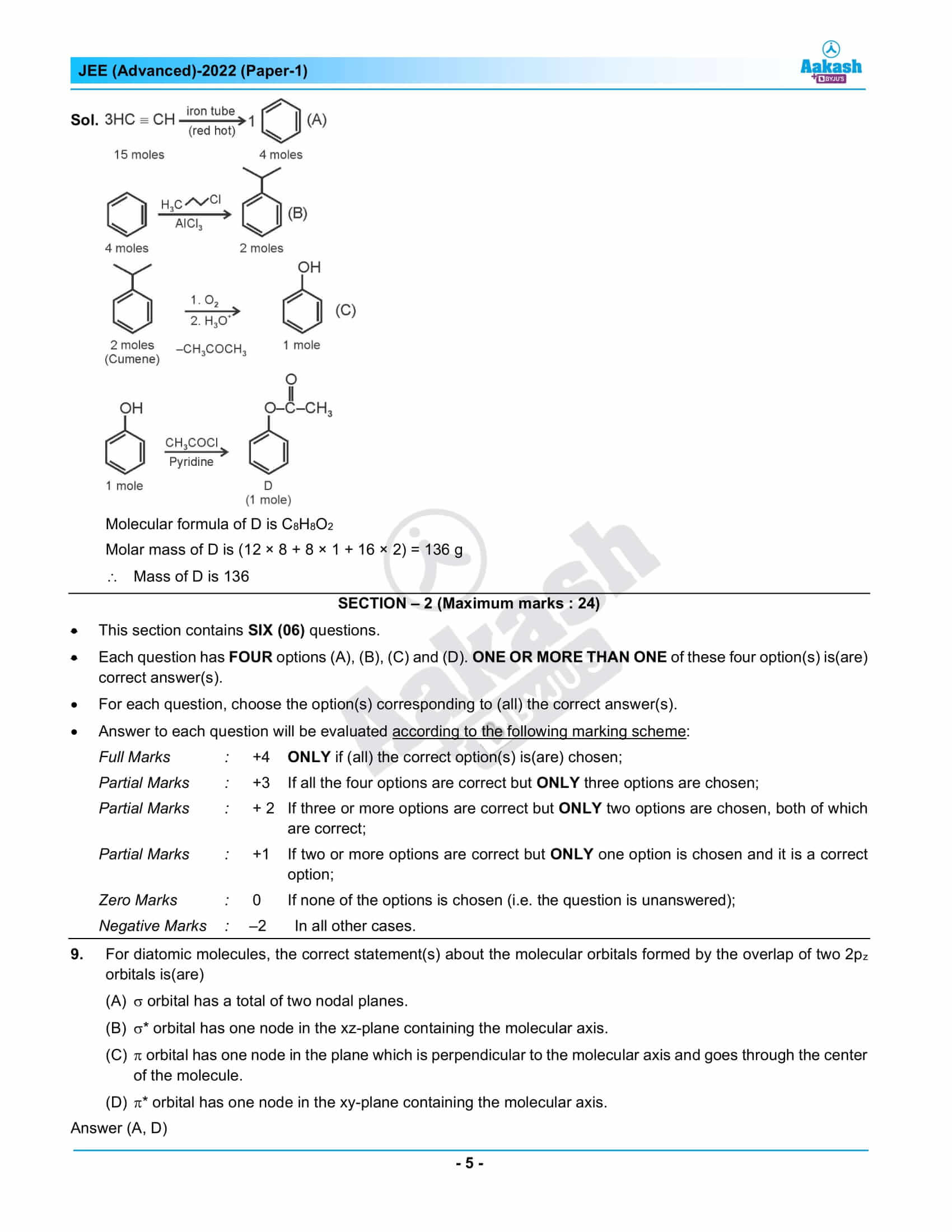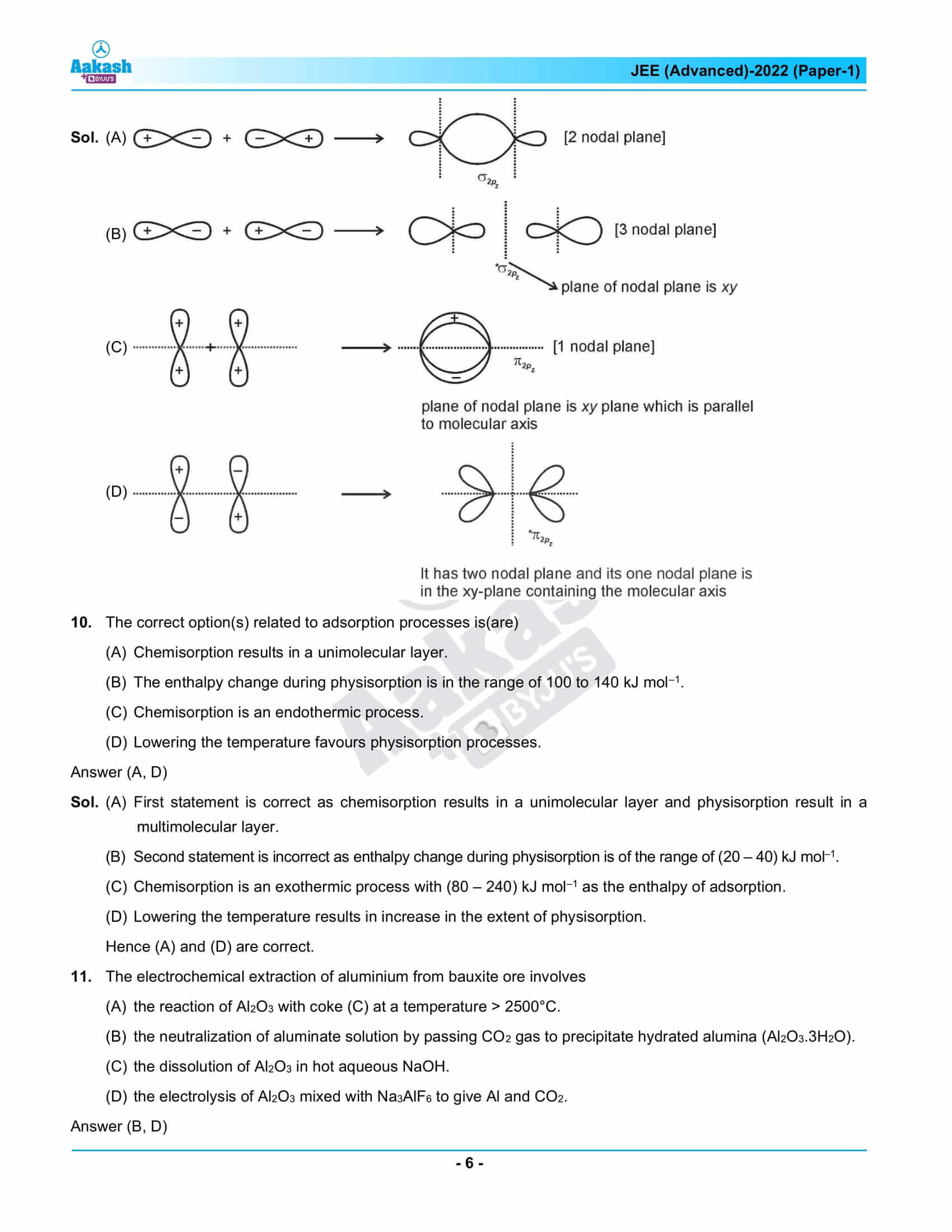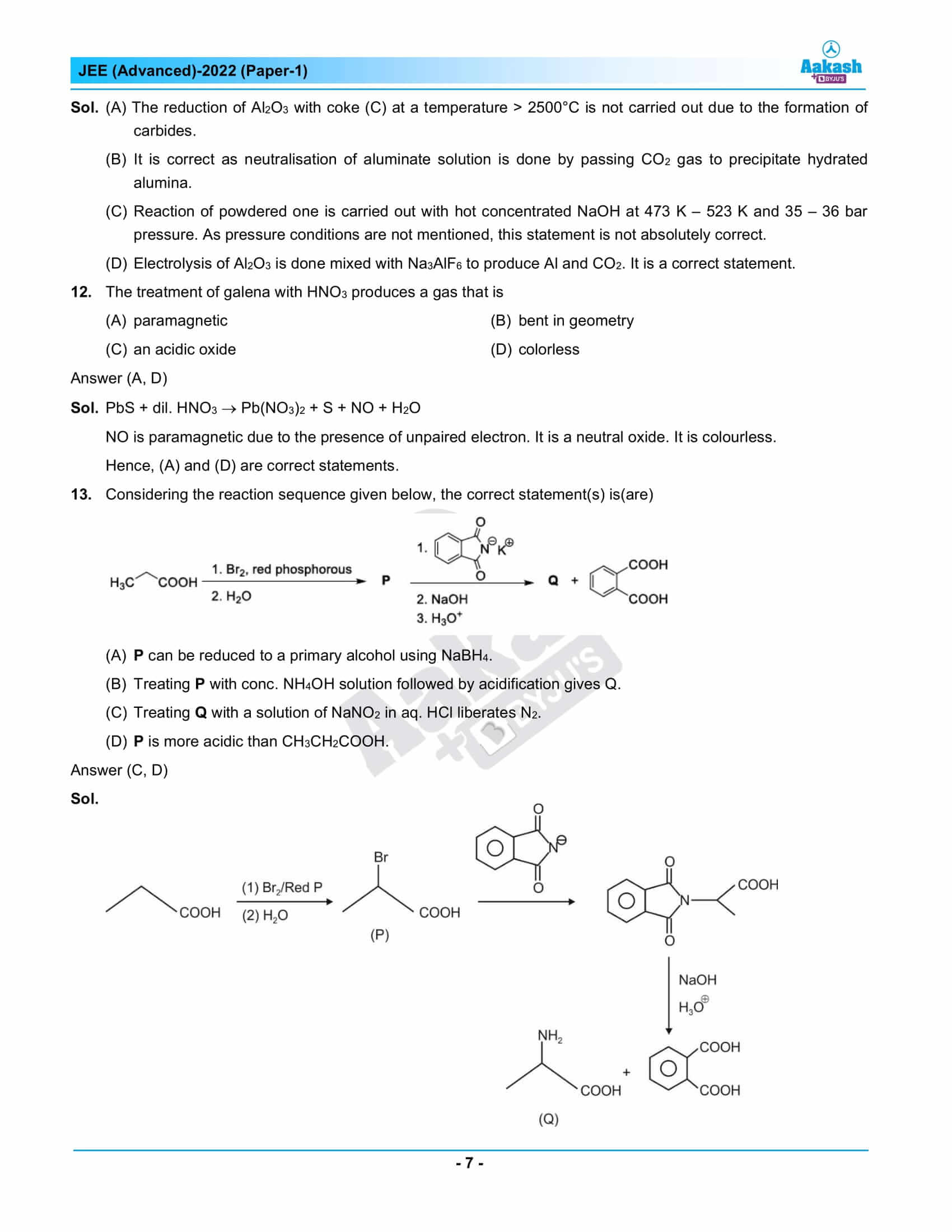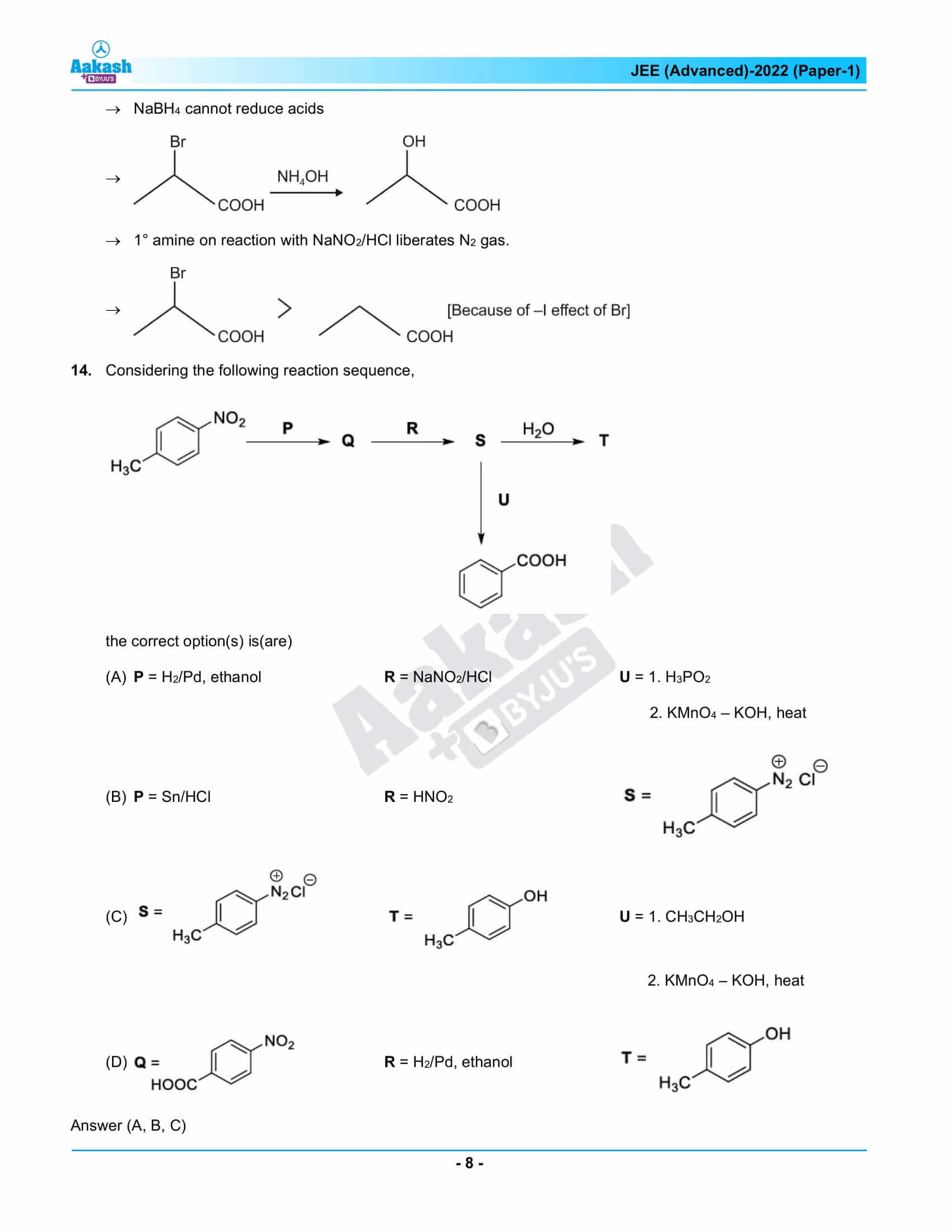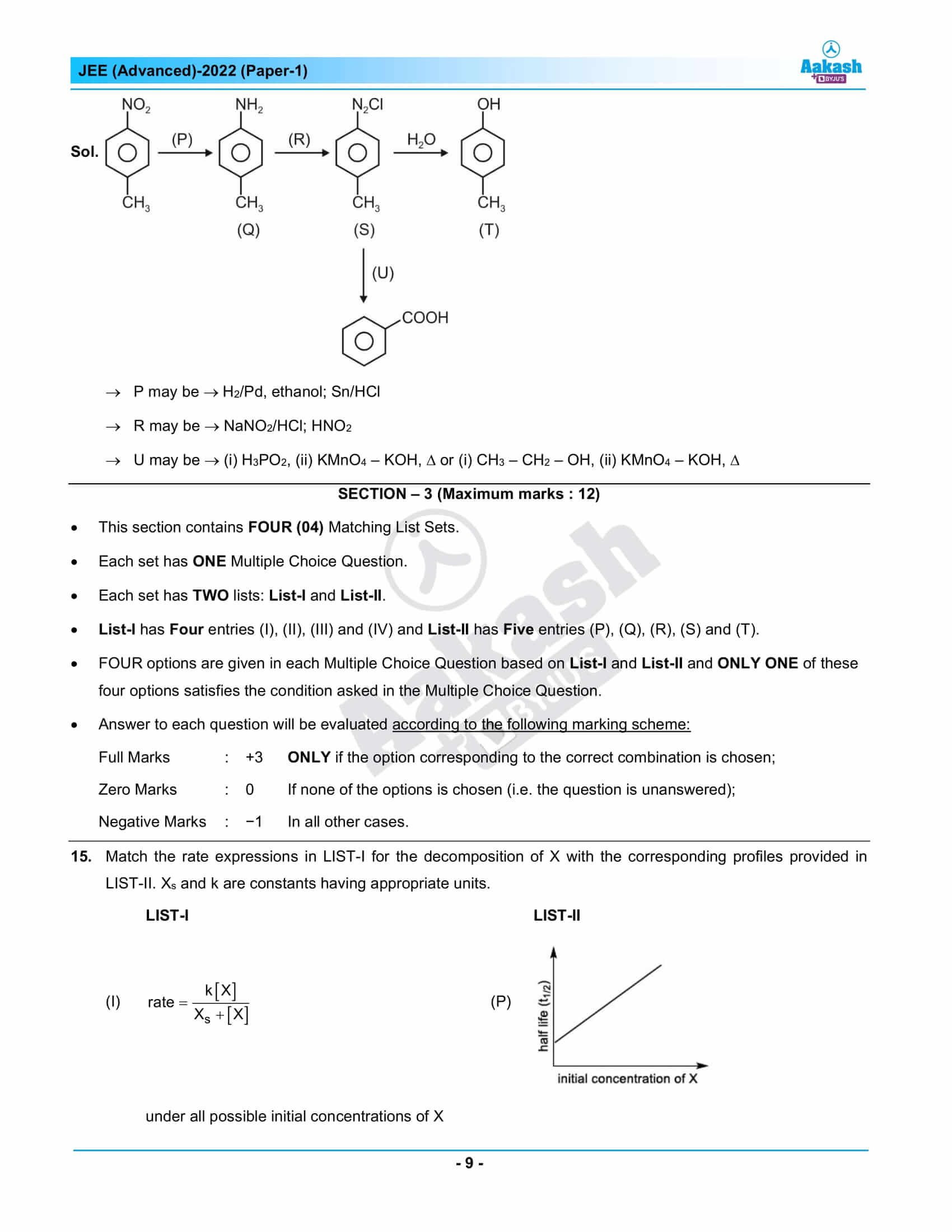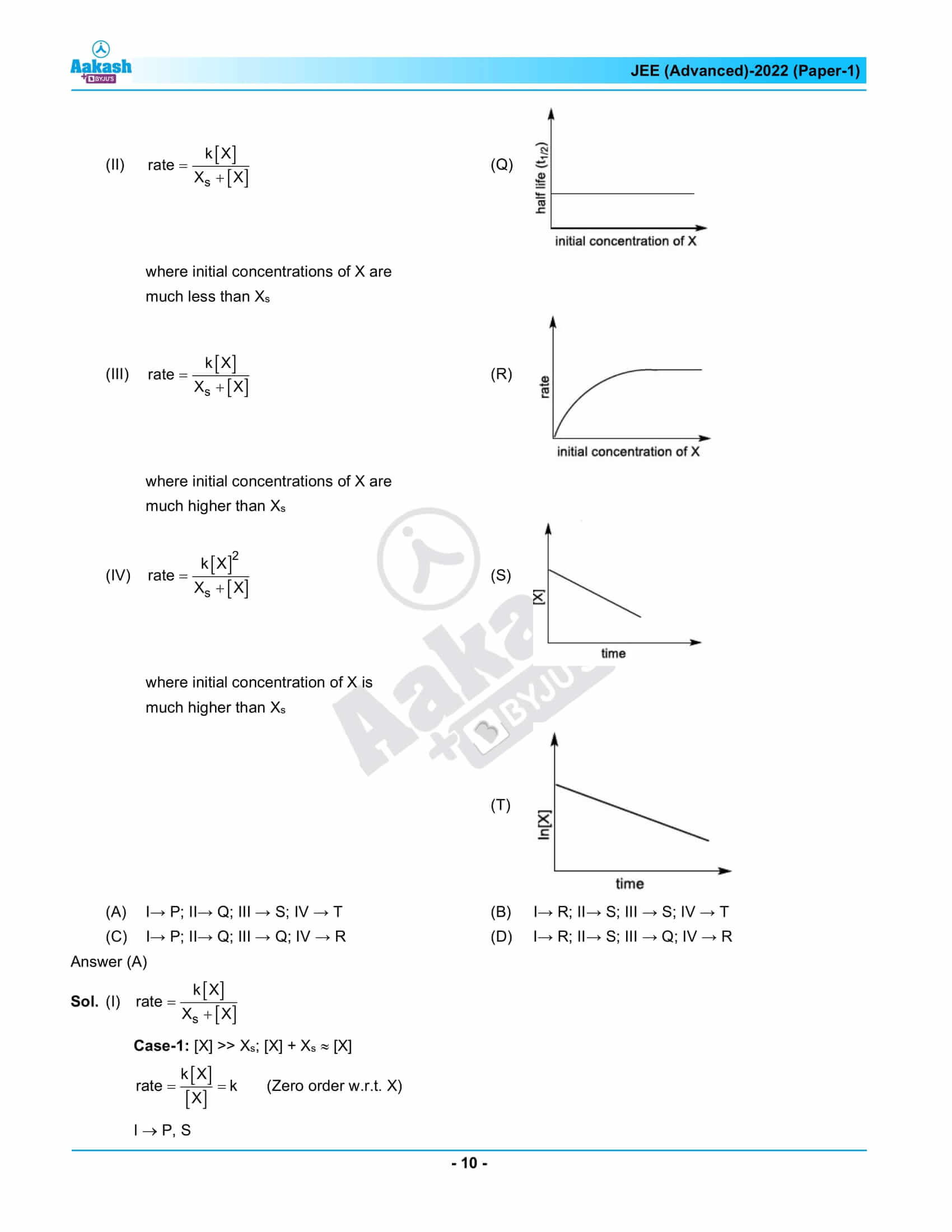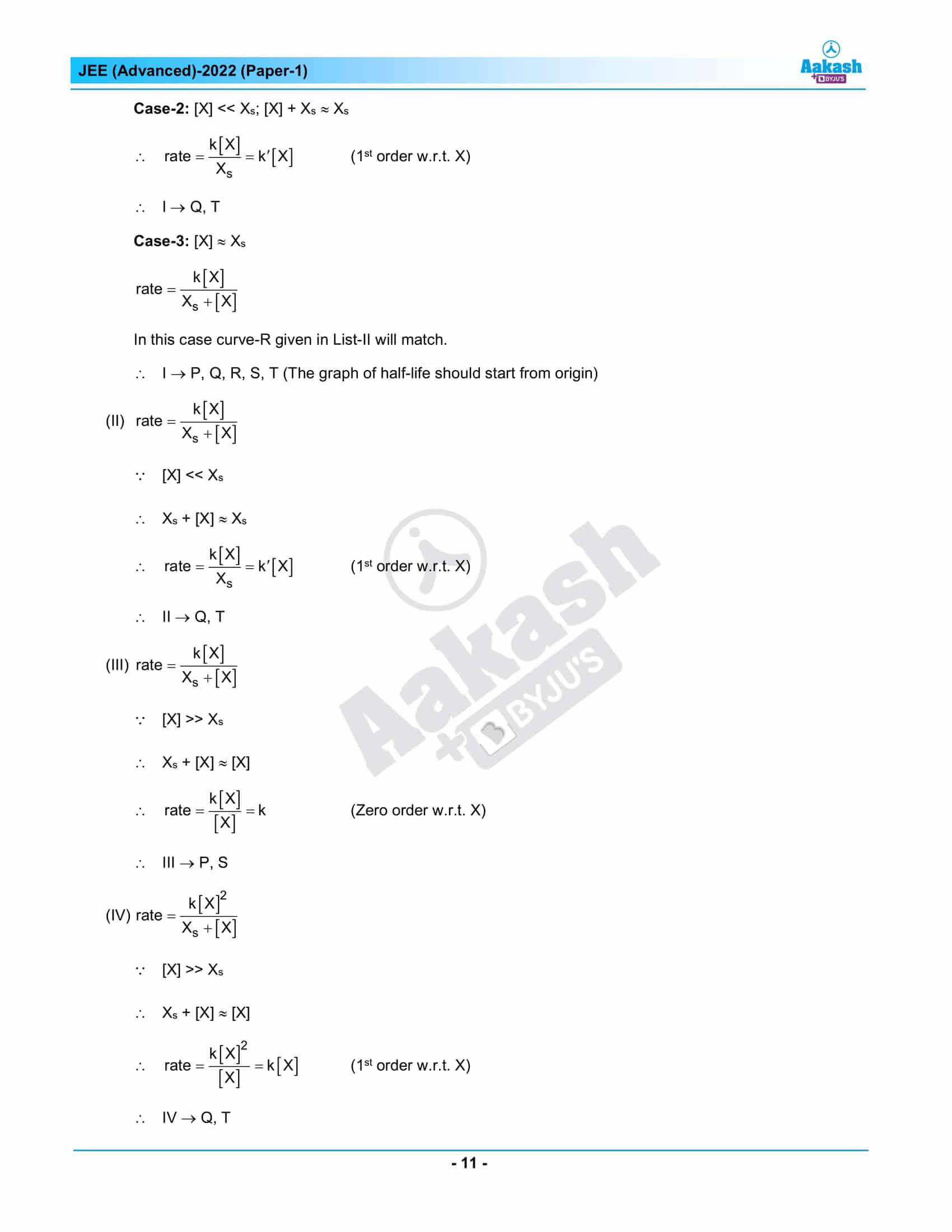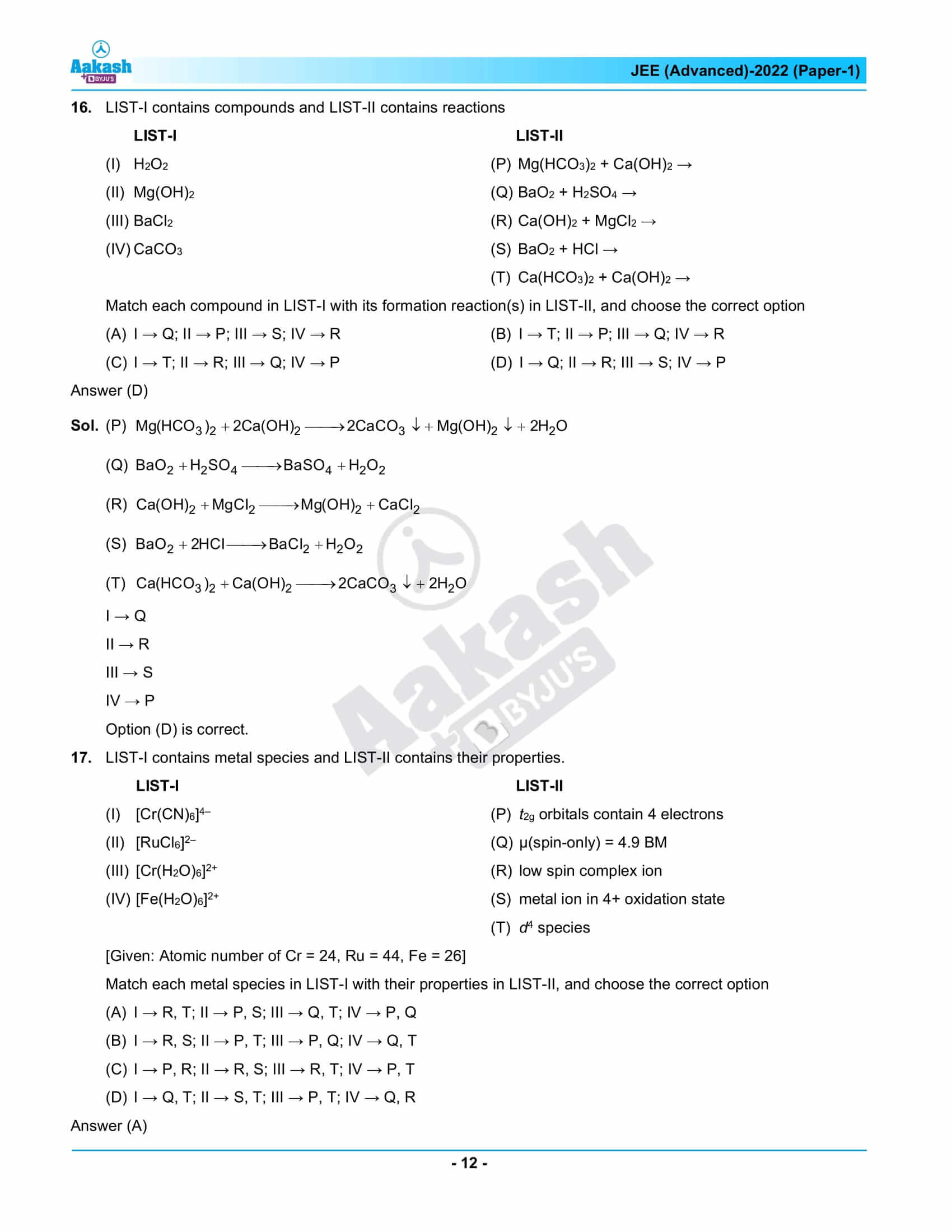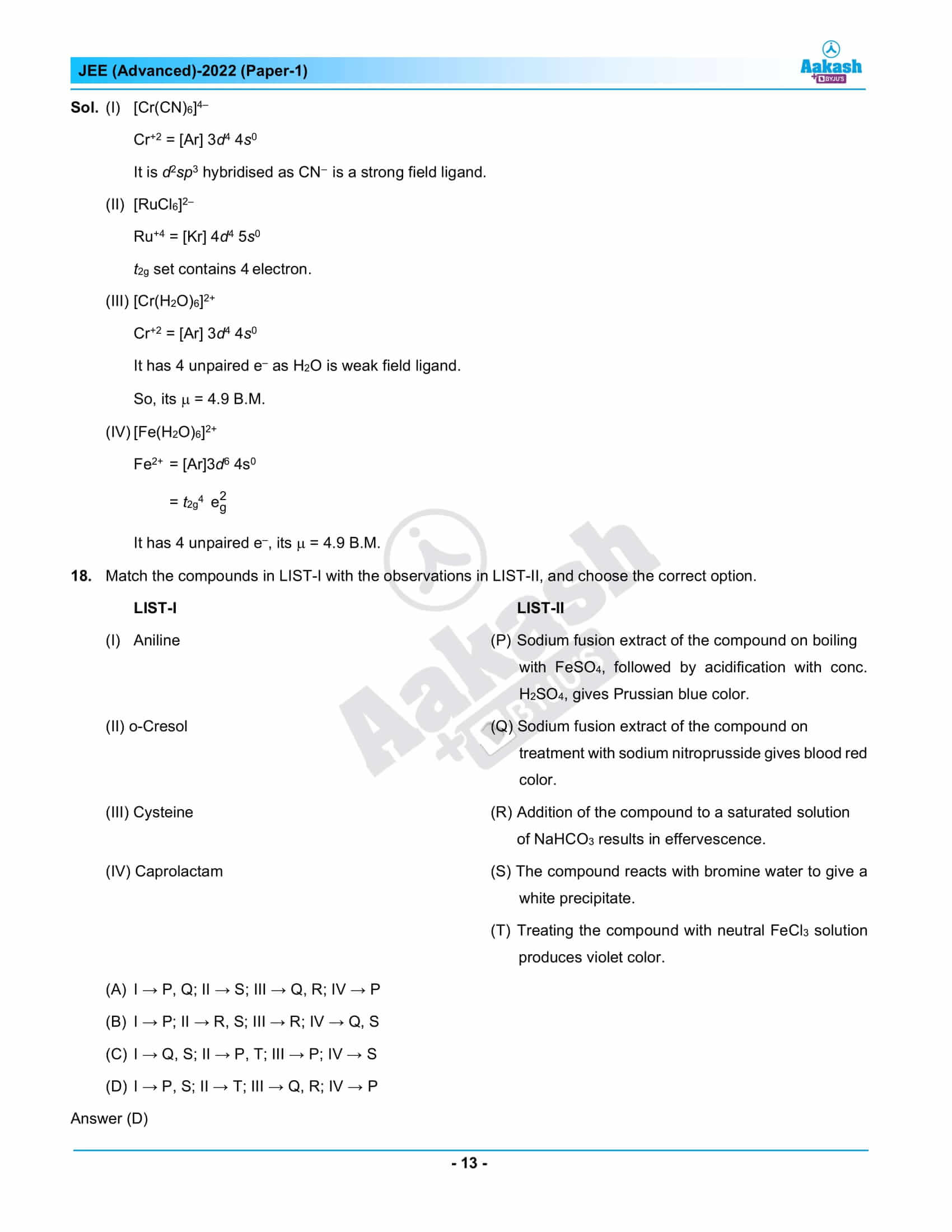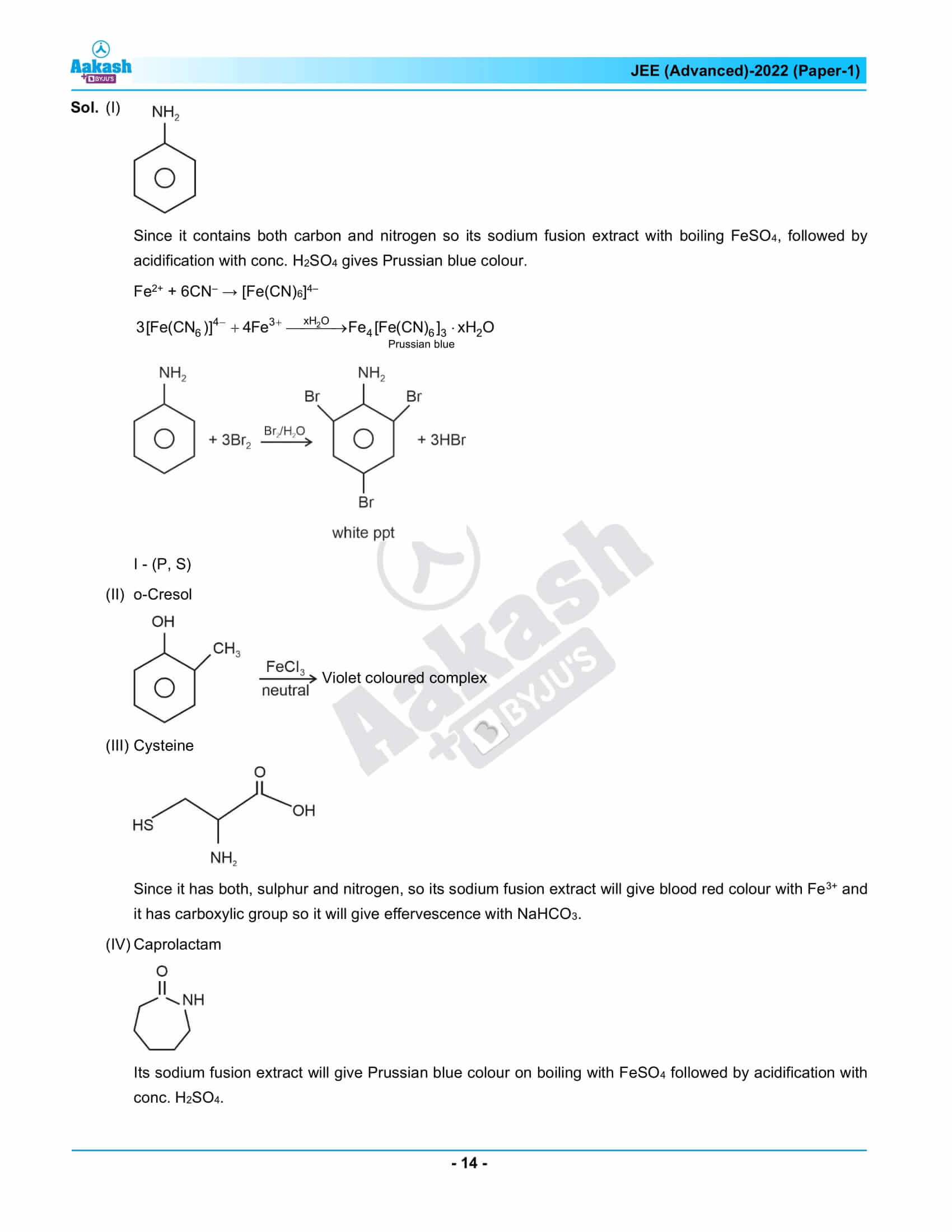## JEE Advanced 2022 Question Paper 1 – Live Paper Analysis and Solutions## Frequently Asked Questions – FAQs

Q1

### What is the pattern of the JEE Advanced 2022 Chemistry Paper 1?

As we know, the pattern of the JEE Advanced 2022 Chemistry Paper 1 changes every year. This year, Chemistry Paper 1 had 3 sections, with a negative marking for a few questions.
Q2

### What is the duration of JEE Advanced 2022 Chemistry Paper 1 examination?

The duration of the Paper 1 examination is 3 hours. The Paper 1 question paper includes three parts: Physics, Chemistry and Mathematics. Hence, no specific time is allotted for JEE Advanced 2022 chemistry paper 1.
Q3

### Were questions asked in JEE Advanced 2022 Chemistry Paper 1 easy to attempt?

JEE Advanced 2022 Chemistry paper 1 had moderate to difficult level of questions.
Q4

### How many tough questions appeared in the Chemistry question paper 1 – JEE Advanced 2022?

Six tough questions appeared in the Chemistry question paper 1 – JEE Advanced 2022.
Q5

### Which chapters of Chemistry had the easy questions in the JEE Advanced 2022 question paper 1?

Surface chemistry and S-block elements had the easy questions in the JEE Advanced 2022 question paper 1.